# Johansen Cointegration Test

In time series analysis, we often encounter situations where we wish to model one non-stationary time series ($Y_t$) as a linear combination of other non-stationary time series ($X_{1,t},X_{2,t},\cdots,X_{k,t}$). In other words:

$$Y_t=\beta_o+\beta_1X_{1,t}+\beta_2X_{2,t}+\cdots+\beta_kX_{k,t}+\epsilon_t$$

In general, a regression model for non-stationary time series variables gives spurious (nonsense) results. The only exception is if the linear combination of the (dependent and explanatory) variables eliminates the stochastic trend and produces stationary residuals.

$$Y_t+\gamma_1X_{1,t}+\gamma_2X_{2,t}+\cdots+\gamma_kX_{k,t}\sim I(0)$$

In this case, we refer to the set of variables as cointegrated. It is only in this case that we can look at regression as a reasonable and reliable model.

In this paper, we’ll discuss one important question:

1. How do we examine a set of non-stationary variables for Cointegration?

In future issues, we’ll tackle the topics of long-run and short-run dynamics of cointegrated time series variables using OLS regression and error correction models.

### Motivation

Cointegration means that, while many developments can cause permanent changes in the individual variable (i.e., $x_{i,t}$), there is some long-run equilibrium relation tying the individual variables together, represented by some linear combination of them.

Why do we care?

Ignoring the cointegration aspect in time series variables may lead to a spurious regression problem, which occurs if arbitrarily trending and/or non-stationary series are regressed on each other.

1. In the case of a deterministic trending series, the spuriously found relationship is due to the trend governing both series instead of the economic forces.
2. In the case of non-stationarity (of type $I(1)$), the series – even without trend – tends to show local trends which tend to co-move along for relatively long periods.

In trading, a trader may buy one security and hedge it with another type of security (e.g. spreads). Such strategies are based on the belief that two securities are somewhat related and that a long-run equilibrium should exist between them.

In economics and finance, academics use cointegrated variables to test plausible economic relationships, under the hypothesis of a long-run equilibrium between non-stationary time series (e.g. disposable income vs. private consumption).

### Background

In a nutshell, cointegration assumes there is a common stochastic non-stationary (i.e. $I(1)$ ) process underlying two (or more) processes X and Y.

$$X_t=\gamma_o+\gamma_1Z_t+\epsilon_t\sim I(1)$$ $$Y_t=\delta_o+\delta_1Z_t+\eta_t\sim I(1)$$ $$Z_t\sim I(1)$$ $$\epsilon_t,\eta_t\sim I(0)$$

$\epsilon,\eta$ are stationary processes ($I(0)$) with zero mean, but they can be serially correlated.

Although $X_t$ and $Y_t$ are both non-stationary ($I(1)$), there exists a linear combination of them, which is stationary:

$$\delta_1X_t-\gamma_1Y_t\sim I(0)$$

In other words, the regression of Y and X yields stationary residuals $\{\epsilon\}$.

In general, given a set of non-stationary (of type $I(1)$) time series variables $\{X_{1,t},X_{2,t},\cdots,X_{k,t}\}$, there exists a linear combination consisting of all variables with a vector $\beta$, such that:

$$\beta_1X_{1,t}+\beta_2X_{2,k}+\cdots+\beta_kX_{k,t}\sim I(0)$$

Where $\beta_j\neq 0, j=1,2,\cdots,k$.If this is the case, then the $X$'s are cointegrated to the order of $C.I(1,1)$.

Testing of Cointegration

In principle, testing for Cointegration is similar to testing the linear regression residuals ($\epsilon_t$) for stationarity.

$$X_{1,t}=\alpha+\beta_2X_{2,k}+\beta_3X_{3,k}+\cdots+\beta_kX_{k,t}+\epsilon_t$$

So, to establish a cointegration relationship, you would run first an OLS regression model for your variables and test the residuals for stationarity.

Sounds simple? It is. But which variable should we select as the dependent variable? Does it matter? It turns out that it does matter.

Why? The residuals vary based on which time series is designated as the dependent variable, and the tests may give different results.

One important test for cointegration that is invariant to the ordering of variables is the full-information maximum likelihood test of Johansen (aka Johansen test).

Johansen Test

The Johansen test approaches the testing for cointegration by examining the number of independent linear combinations (k) for an m time series variables set that yields a stationary process.

Why?

Early in this paper, we stated that cointegration assumes the presence of common non-stationary (i.e. $I(1)$) processes underlying the input time series variables.

$$X_{1,t}=\alpha_1+\gamma_1Z_{1,t}+\gamma_2Z_{2,t}+\cdots+\gamma_pZ_{p,t}+\epsilon_{1,t}$$ $$X_{2,t}=\alpha_2+\phi_1Z_{1,t}+\phi_2Z_{2,t}+\cdots+\phi_pZ_{p,t}+\epsilon_{2,t}$$ $$\cdots$$ $$X_{m,t}=\alpha_m+\psi_1Z_{1,t}+\psi_2Z_{2,t}+\cdots+\psi_pZ_{p,t}+\epsilon_{m,t}$$

The number of independent linear combinations (k) is related to the assumed number of common non-stationary underlying processes (p) as follows:

$$p=m-k$$

So, let’s consider three plausible outcomes:

1. $k=0,p=m$. In this case, time series variables are not cointegrated
2. 0 < k < m, 0 < p < m. In this case, the time series variables are cointegrated.
3. $k=m,p=0$. All time-series variables are stationary ($I(0)$ to start with. Cointegration is not relevant here.

By examining the number of independent combinations, we are indirectly examining the cointegration existence hypothesis.

The Johansen test has two forms: the trace test and the maximum eigenvalue test. Both forms/tests address the Cointegration presence hypothesis, but each asks very different questions.

Trace Test

The trace test examines the number of linear combinations (i.e. $K$ ) to be equal to a given value ($K_o$), and the alternative hypothesis for $K$ to be greater than $K_o$

$$H_o:K=K_o$$ $$H_1:K > K_o$$

To test for the existence of Cointegration using the trace test, we set $K_o=0$ (no cointegration), and examine whether the null hypothesis can be rejected. If this is the case, then we conclude there is at least one cointegration relationship.

In this case, we need to reject the null hypothesis to establish the presence of Cointegration between the variables.

Maximum Eigenvalue Test

With the maximum eigenvalue test, we ask the same central question as in the Johansen test. The difference, however, is an alternate hypothesis:

$$H_o:K=K_o$$ $$H_1:K = K_o+1$$

So, starting with $K_o=0$ and rejecting the null hypothesis implies that there is only one possible combination of the non-stationary variables to yield a stationary process. What if we have more than one? The test may be less powerful than the trace test for the same $K_o$ values.

A special case for using the maximum eigenvalue test is when $K_o=m-1$, where rejecting the null hypothesis implies the existence of m possible linear combinations. This is impossible unless all input time series variables are stationary ($i(0)$) to start with.

In NumXL, the Johansen test combines these two test forms to examine the cointegration assumption:

• Trace Test for $K_o=0$.
• Maximum Eigenvalue Test for $K_o=m-1$.

To establish the existence of cointegration in a set of time series variables, we wish to reject the trace test null hypothesis ($K_o=0$) and not reject the null hypothesis of the maximum eigenvalue test ($K_o=m-1$).

## Process

Now, let’s go over the steps for conducting a cointegration test in NumXL.

1. Organized your input time series data as adjacent columns. Each column represents one variable and each row corresponds to an observation.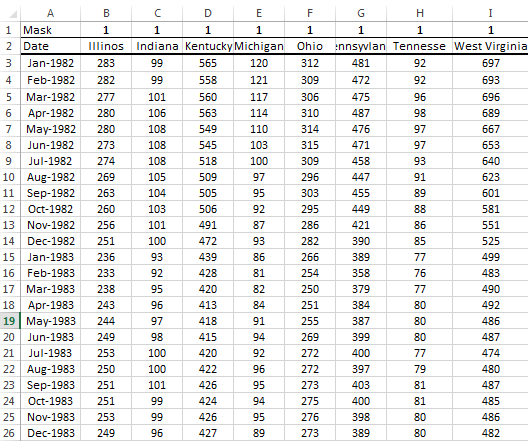2. Locate the cointegration test icon in the NumXL menu or toolbar and click on it.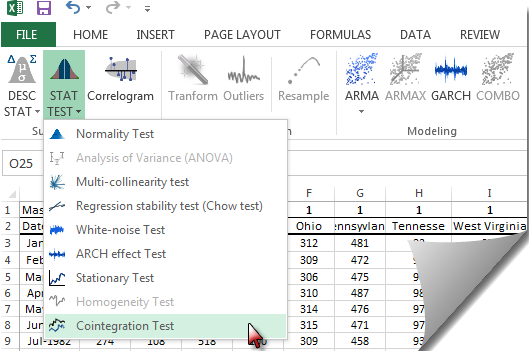3. Using the cointegration wizard, select your input variables. The selection may include column labels.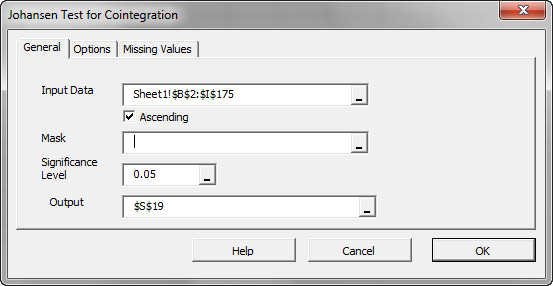Note: The “Mask” field is used to exclude variables/columns from the analysis without changing your input data in the worksheet. In our tutorial, we want to include all of them, so we can leave it blank.
After we select the input data, the “Options” and “Missing Values” tabs are enabled.
4. Initially, all Johansen tests are selected and a maximum lag order is calculated from the input data, but you can override any of those options as you see fit.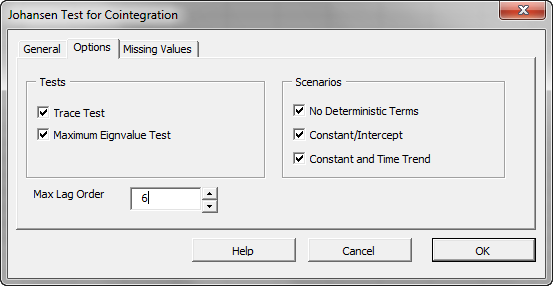Let’s leave it unchanged.
5. (Optional) If your input data does not have any missing values, you may skip this step.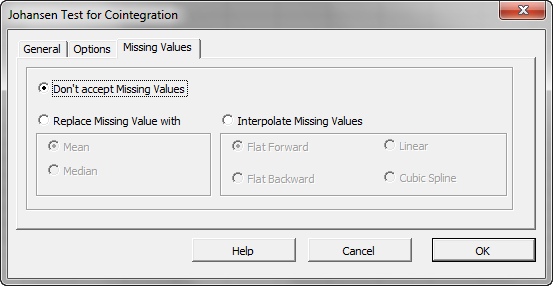By default, the cointegration wizard will trigger an error if any of the variables has a missing value. This is acceptable for this tutorial.
6. Click the “OK” button.

## Output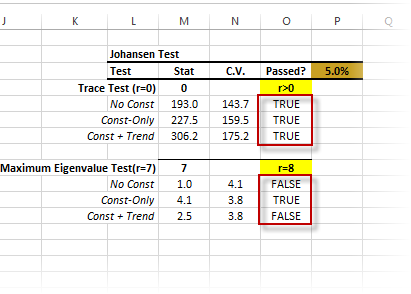When examining the output tables, keep this in mind:

• Under the trace test, we asked whether there’s at least one possible linear combination for the input variables to yield a stationary process. We examined this question under the different assumptions for the input variable, and they all passed. Thus, we can conclude that the variables are cointegrated.
• Next, under the maximum eigenvalue test, we want to be sure that the number of linear combinations does not equal the number of input variables. Why? Because if they do, the input variables are stationary to start with, and cointegration is not relevant. Again, we carry on the test under different assumptions for the input variables. In this example, they all failed the test aside from one scenario, which passed marginally.

In conclusion, we would state that the input variables are cointegrated.

Now what? You may use OLS regression for one variable using the other variables without the risk of getting into a spurious regression problem.